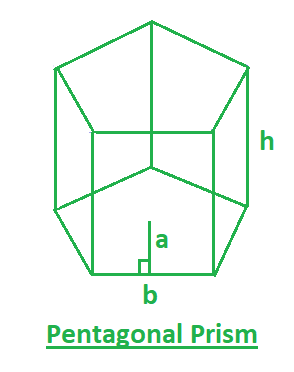# Program to find volume and surface area of pentagonal prism

A prism that has 5 rectangular faces and 2 parallel pentagonal bases is a pentagonal prism. So you are given the apothem length(a), base length(b) and height(h) of the pentagonal prism. you have to find the surface area and the volume of the Pentagonal Prism.

Examples:

Input : a=3, b=5, h=6
Output :surface area=225, volume=225

Input : a=2, b=3, h=5
Output :surface area=105, volume=75In this figure,
a– apothem length of the Pentagonal Prism.
b– base length of the Pentagonal Prism.
h– height of the Pentagonal Prism.

Formulas:Below are the formulas for calculating the surface area and the volume of the Pythagonal Prism.## C++

 // CPP program to find  // surface area and volume of the  // Pentagonal Prism  #include  using namespace std;     // function for surface area  float surfaceArea(float a, float b, float h)  {      return 5 * a * b + 5 * b * h;  }     // function for VOlume  float volume(float b, float h)  {      return (5 * b * h) / 2;  }     // Driver function  int main()  {      float a = 5;      float b = 3;      float h = 7;         cout << "surface area= " << surfaceArea(a, b, h) << ", ";         cout << "volume= " << volume(b, h);  }

## Java

 // Java program to find  // surface area and volume of the  // Pentagonal Prism  import java.util.*;     class solution  {     // function for surface area  static float surfaceArea(float a, float b, float h)  {         return 5 * a * b + 5 * b * h;     }     // function for VOlume  static float volume(float b, float h)  {         return (5 * b * h) / 2;     }     // Driver function  public static void main(String arr[])  {      float a = 5;      float b = 3;      float h = 7;         System.out.println( "surface area= "+surfaceArea(a, b, h)+", ");         System.out.println("volume= "+volume(b, h));  }  }

## Python3

 # Python 3 program to find surface area  # and volume of the Pentagonal Prism     # function for surface area  def surfaceArea(a, b, h):      return 5 * a * b + 5 * b * h     # function for VOlume  def volume(b, h):      return (5 * b * h) / 2    # Driver Code  if __name__ == '__main__':      a = 5     b = 3     h = 7        print("surface area =", surfaceArea(a, b, h),                     ",", "volume =", volume(b, h))     # This code is contributed by  # Sanjit_Prasad

## C#

 // C# program to find surface  // area and volume of the  // Pentagonal Prism  using System;     class GFG  {     // function for surface area  static float surfaceArea(float a,                            float b, float h)  {      return 5 * a * b + 5 * b * h;  }     // function for VOlume  static float volume(float b, float h)  {      return (5 * b * h) / 2;  }     // Driver Code  public static void Main()  {      float a = 5;      float b = 3;      float h = 7;         Console.WriteLine("surface area = " +               surfaceArea(a, b, h) + ", ");         Console.WriteLine("volume = " +                       volume(b, h));  }  }     // This code is contributed by vt_m

## PHP

 

Output:

surface area= 180, volume= 52.5


My Personal Notes arrow_drop_upCheck out this Author's contributed articles.

If you like GeeksforGeeks and would like to contribute, you can also write an article using contribute.geeksforgeeks.org or mail your article to contribute@geeksforgeeks.org. See your article appearing on the GeeksforGeeks main page and help other Geeks.

Please Improve this article if you find anything incorrect by clicking on the "Improve Article" button below.